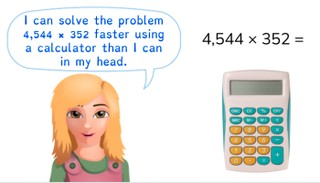Multiplying and dividing larger numbers with the calculator

# Multiplying and dividing larger numbers with the calculator

Students learn to multiply and divide larger numbers with a calculator.8,000 schools use Gynzy92,000 teachers use Gynzy1,600,000 students use Gynzy

## General

The students learn to multiply and divide larger numbers with the calculator.

## Standards

CCSS.Math.Practice.MP5

## Learning objective

Students will be able to multiply and divide larger numbers using a calculator.

## Introduction

Click on the speaker to have the number spoken. Then have the students write down each number that they hear.

## Instruction

During this lesson, the students can follow along by solving problems with their calculator. Show that numbers greater than 1,000 don't have a comma on the calculator, but that you should put one in your answer when writing it down. Practice the following problems using the calculator. Emphasize that you should always include a comma where it is needed when writing a number. Then practice a division problem on the calculator. Walk through the tips for using a calculator with the students. Next the students can solve the multiplication and division problems on their own. Remind the students how they should write numbers in the millions. To show this, use the example of 0.3 million. Practice writing the following three numbers together with the students. Next you have the students solve four more multiplication and division problems, for which they should write down the answer with a comma if needed. Finally there is both a multiplication problem and a division problem in story form.

Check whether the students can multiply and divide larger numbers with the calculator, by asking the following question:
- Why is it important to be able to multiply and divide large numbers with the calculator?

## Quiz

The students test their understanding of multiplying and dividing larger numbers with the calculator through ten exercises. Some of the exercises are multiplication problems, and others are division problems. Some exercises are story problems.

## Closing

Discuss once again the importance of being able to multiply and divide larger numbers with the calculator. As a closing activity the students can work in pairs to solve the math problem.

## Teaching tips

Have students that have difficulty with multiplying and dividing larger numbers with the calculator first practice typing numbers correctly in a calculator. Make sure they are using the right digits and symbols. Emphasize that the calculator does not have commas, but when writing numbers greater than 1,000, you still have to include them.

## Instruction materials

Calculators.

### The online teaching platform for interactive whiteboards and displays in schools

• Save time building lessons

• Manage the classroom more efficiently

• Increase student engagement